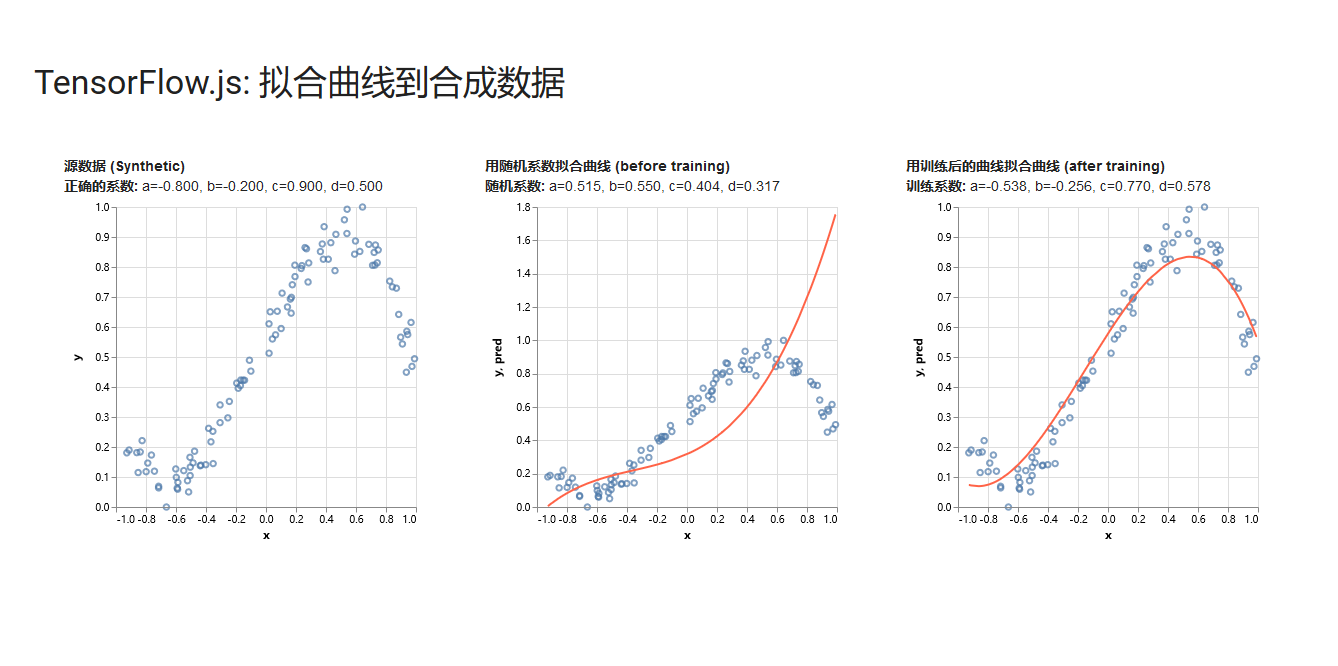# Tensorflow.js

TensorFlow.js 是一个开源库，不仅可以在浏览器中运行机器学习模型，还可以训练模型。

TensorFlow.js 对未来 web 开发有着重要的影响，JS 开发者可以更容易地实现机器学习，工程师和数据科学家们可以有一种新的方法来训练算法，例如官网上 Emoji Scavenger Hunt 这样的游戏界面，让用户一边玩游戏一边将模型训练地更好。

# 官方案例—tensorflowjs拟合曲线

$git clone https://github.com/tensorflow/tfjs-examples$ cd tfjs-examples/polynomial-regression-core
$yarn$ yarn watch
• 第1步：设置变量
首先，我们需要创建一些变量。即开始我们是不知道a、b、c、d的值的，所以先给他们一个随机数，入戏所示：
const a = tf.variable(tf.scalar(Math.random()));
const b = tf.variable(tf.scalar(Math.random()));
const c = tf.variable(tf.scalar(Math.random()));
const d = tf.variable(tf.scalar(Math.random()));

• 第2步：建立模型
function predict(x) {
// y = a * x ^ 3 + b * x ^ 2 + c * x + d
return tf.tidy(() => {
return a.mul(x.pow(tf.scalar(3))) // a * x^3
.add(b.mul(x.square())) // + b * x ^ 2
.add(c.mul(x)) // + c * x
.add(d); // + d
});
}
• 第3步：训练模型
最后一步就是要训练这个模型使得系数和这些散点更加匹配，而为了训练模型，我们需要定义下面的三样东西：

• 定义损失函数
这篇文章中，我们使用MSE（均方误差，mean squared error）作为我们的损失函数。MSE的计算非常简单，就是先根据给定的x得到实际的y值与预测得到的y值之差 的平方，然后在对这些差的平方求平均数即可。
$MSE=\left(y-{y}^{{}^{\prime }}{\right)}^{2}$
function loss(predictions, labels) {
// 将labels（实际的值）进行抽象
// 然后获取平均数.
const meanSquareError = predictions.sub(labels).square().mean();
return meanSquareError;
}
• 定义优化器

TensorFlow.js提供了一个很方便的函数用来实现SGD，所以你不需要担心自己不会这些特别复杂的数学运算。 即 tf.train.sdg 将一个学习率（learning rate）作为输入，然后返回一个SGDOptimizer对象，它与优化损失函数的值是有关的。

const learningRate = 0.5;
const optimizer = tf.train.sgd(learningRate);
• 定义训练循环

function train(xs, ys, numIterations = 75) {

const learningRate = 0.5;
const optimizer = tf.train.sgd(learningRate);

for (let iter = 0; iter < numIterations; iter++) {
optimizer.minimize(() => {
const predsYs = predict(xs);
return loss(predsYs, ys);
});
}
• 实验结果